A particle of mass m is confined in the poten...

### Related TestA particle of mass m is confined in the potential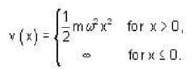Let the wave function of the particle be given by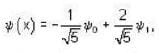where y0 and y1 are the eigen functions of the ground state and the first excited state respectively. The expectation value of the energy is
• a)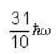• b)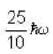• c)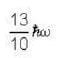• d)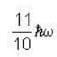Saroj Devi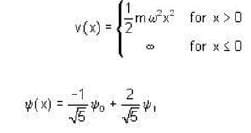y0 ground state eigen function y,® first excited state eigen function energy eigen value E =(n+1/2)ℏω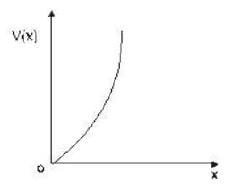for ground state n = 0, E =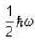for first excited state n =1, E =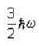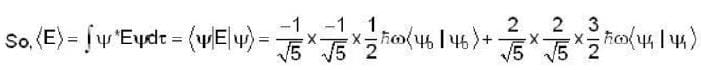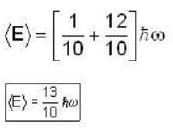View courses related to this question Explore GATE courses
 Explore GATE coursesView courses related to this question1 Crore+ students have signed up on EduRev. Have you?

• ### How many of the following statements are true?1) Function must be neutral f... more(Scan QR code)